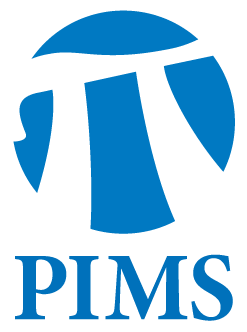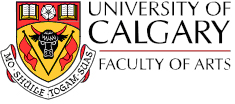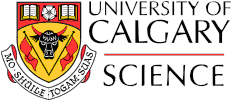## Alan Turing Centenary 2012: Lecture by Dr. Richard Zach, University of Calgary

• Date: 01/24/2012
Lecturer(s):
Dr. Richard Zach, Department of Philosophy, University of Calgary
Location:

University of Calgary

Topic:

Alan Turing and the Decision Problem

Description:

Many scientific questions are considered solved to the best possible degree when we have a method for computing a solution.  This is especially true in mathematics and those areas of science in which phenomena can be described mathematically: one only has to think of the methods of symbolic algebra in order to solve equations, or laws of physics which allow one to calculate unknown quantities from known measurements.  The crowning achievement of mathematics would thus be a systematic way to compute the solution to any mathematical problem.  The hope that this was possible was perhaps first articulated by the 18th century mathematician-philosopher G. W. Leibniz. Advances in the foundations of mathematics in the early 20th century made it possible in the 1920s to first formulate the question of whether there is such a systematic way to find a solution to every mathematical problem. This became known as the decision problem, and it was considered a major open problem in the 1920s and 1930s.  Alan Turing solved it in his first, groundbreaking paper "On computable numbers" (1936).  In order to show that there cannot be a systematic computational procedure that solves every mathematical question, Turing had to provide a convincing analysis of what a computational procedure is. His abstract, mathematical model of computability is that of a Turing Machine. He showed that no Turing machine, and hence no computational procedure at all, could solve the Entscheidungsproblem.

Other Information:

Location:  ICT 122

Additional information can be viewed here: